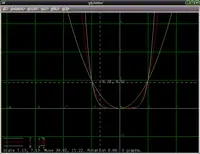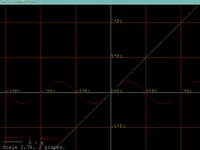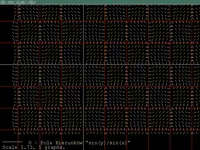# glplotter (version 2.0.0)glplotter draws graphs of mathematical functions. You specify a mathematical expression to be used as a function, using a straightforward syntax, like "`sin(x) + x^2`".

glplotter can also load a graph from a file. This is useful if your graph data doesn't come from any mathematical expression — e.g. maybe it's some collected statistical data. Maybe your data is not even a function at all — actually any shape consisting of line segments may be displayed by glplotter.

Documentation:

## 1. Command-line parameters

You can pass at command-line file names from which to load graphs. Dash (`-`) as filename means "standard input".

E.g. you could pipe the output of `gen_function` program to glplotter, like

```  gen_function "sin(x)" -10 10 0.1 | glplotter -
```
which will display the graph of sinus for X in [-10; 10]. Commands like
```  gen_function "sin(x)" -10 10 0.1 > plot.1
gen_function "x^2" -10 10 0.1 > plot.2
glplotter plot.1 plot.2
```
will display graphs of sinus and x2 at once. Of course, in case of function expressions, it's usually more comfortable to set them up inside glplotter using "Functions" menu.

Options `--light` and `--dark` allow you to choose appropriate color scheme.

Option `--custom-size SIZE` (or `-c SIZE`) specifies size for

• grid shown when `--grid-custom` was used (or Ctrl + G pressed)
• numbers scale shown when `--num-scale-custom` was used (or Ctrl + S pressed)
• numbers shown when `--numbers-custom` was used (or Ctrl + N pressed)

## 2. Graph file format

Graph for glplotter is actually just a set of line segments. They don't have to correspond to any function — they can show any shape, they can cross each other etc.

File format:

• Lines starting with `#` (hash) are comments.
• Lines with two float numbers (separated by any whitespae) represent another point of the graph. You can use decimal or scientific float format (like `3.14` or `10e-3`). A line segment will be drawn from this point to the next one (unless a `break` will occur).
• Line with only `break` word means a break in a line segment sequence.
• Line like `name=<graph_name>` specifies graph name (will be used in glplotter legend).
Whitespace at the beginning and end of the line is always ignored.

## 3. gen_function

`gen_function` generates graph file from given function expression. It's seldom needed — glplotter can make a graph from function expression on it's own, see menu "Functions".

Call it like:

```  gen_function <function> <x1> <x2> <xstep>
```

This will write on standard output the graph of function `<function>` for x in range `[<x1> ; <x2>]` (with x sampled at each `<xstep>` distance). The graph will be in format understood by glplotter.

For example

```  gen_function "x^2" 0 5 1
```
will write
```# File generated by gen_function on 14-3-2004 at 23:34:37
#   function = x^2
#   x1 = 0
#   x2 = 5
#   xstep = 1
name=x^2
0.0000000000000000E+0000  0.0000000000000000E+0000
1.0000000000000000E+0000  1.0000000000000000E+0000
2.0000000000000000E+0000  4.0000000000000000E+0000
3.0000000000000000E+0000  9.0000000000000000E+0000
4.0000000000000000E+0000  1.6000000000000000E+0001
5.0000000000000000E+0000  2.5000000000000000E+0001
```

## 4. Syntax of mathematical expressions

Short overview of mathematical expressions syntax: this is really just normal syntax of mathematical expressions, as used in all software and resembling normal mathematical notation. When we deal with function expressions, then `x` represents the argument, e.g. `(x+4)*3+2`, `sin(x)` etc.

For detailed information about syntax and built-in functions, see CastleScript language reference. We use a subset of CastleScript syntax, allowing only a simple expression as a function expression, operating on argument "X".